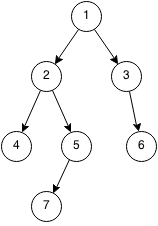## Introduction

Prerequisites: Recursion, Queue

A breadth first search is a search that traverses level by level. For example, in a tree, the search will transverse everything from the first layer, to the second layer, the third layer and all the way down to the last layer. BFS is implemented with a queue.

1. Push the root into the queue.
2. Pop the first element from the queue and push its non-visited neighbours.
3. Repeat 2 until the queue is empty.## Implementation

Printing a binary tree using BFS:

```void bfs(Node root) {
Queue<Node> q = new Queue<Node>();
q.push(root);
while (q.isEmpty() == false) {
Node cur = q.pop();
System.out.println(cur.value);
if (cur.left) {
q.push(cur.left);
}
if (cur.right) {
q.push(cur.right);
}
}
}
```

## Exercises

1. Given a grid of squares with walls at certain locations and two locations A and B, find the minimum distance (going up/left/right/down) between the locations or impossible otherwise. For example if A is at (1,1) and B is at (3,1) but there is a wall at (2,1) then the minimum distance would be 4 (down, left, left, up).
2. Given a tree of letters (A is the root), output the tree using BFS with separators between levels:
• Example: A→B, B→D,B→C, C->G will output A | B | C D | G.
3. Given a tree of letters, and two letters X and Y determine if X is an ancestor of Y or if Y is a ancestor of X or neither. X is an ancestor of Y if X's subtrees contain Y.
• Example: A→B, B→C, B→D, D→G, A is a parent of both C and D but G and C are not ancestors of each other
4. Given a binary tree and a node in the binary tree, find the next node in BFS order of the tree.